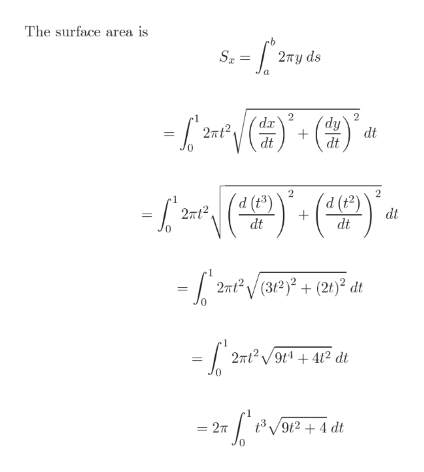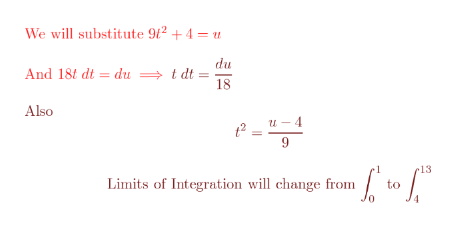# find the exact area of the surface obtained by rotating x=t^3,y=t^2, 0<t<1 about the x-axis.

Question
5 views

find the exact area of the surface obtained by rotating x=t^3,y=t^2, 0<t<1 about the x-axis.

check_circle

Step 1

y=t^2 so t= sqrt(y)

x=t^3= (sqrt(y))^3 = y^(3/2)

x=y^(3/2) (graphed this equation)

Step 2

Use the surface area formula .

There plug x=t^3 and y=t^2 then simplify.help_outlineImage TranscriptioncloseThe surface area is S 2Ty ds 2 'dy da 2nt2 dt dt dt 2 (d(t3) (d (2) 2Tt2 dt dt dt 2nt2/(32 + (20)? dt 2mt2 /9¢* + 4? dt t3 912+4 dt - 2 T fullscreen
Step 3

When t=0 , then u=4

When t=1 then u=1...help_outlineImage TranscriptioncloseWe will substitute 942 +4 = u du And 18t dt = du t dt 18 Also u4 t2 9 e13 Limits of Integration will change from to fullscreen

### Want to see the full answer?

See Solution

#### Want to see this answer and more?

Solutions are written by subject experts who are available 24/7. Questions are typically answered within 1 hour.*

See Solution
*Response times may vary by subject and question.
Tagged in

### Calculus